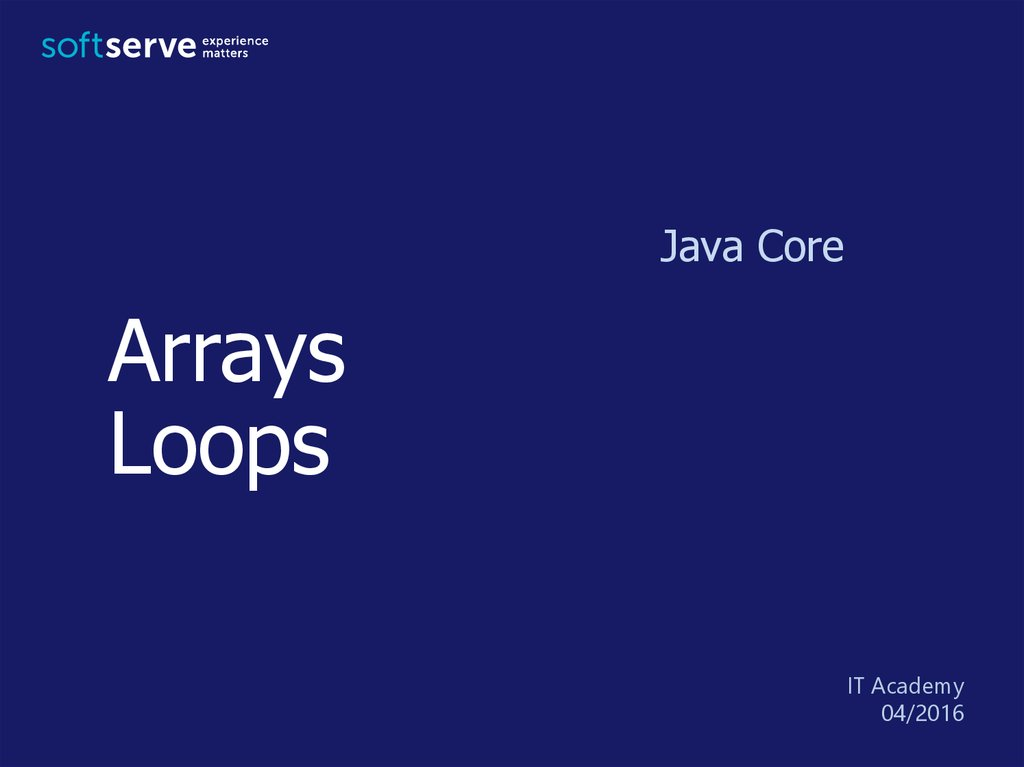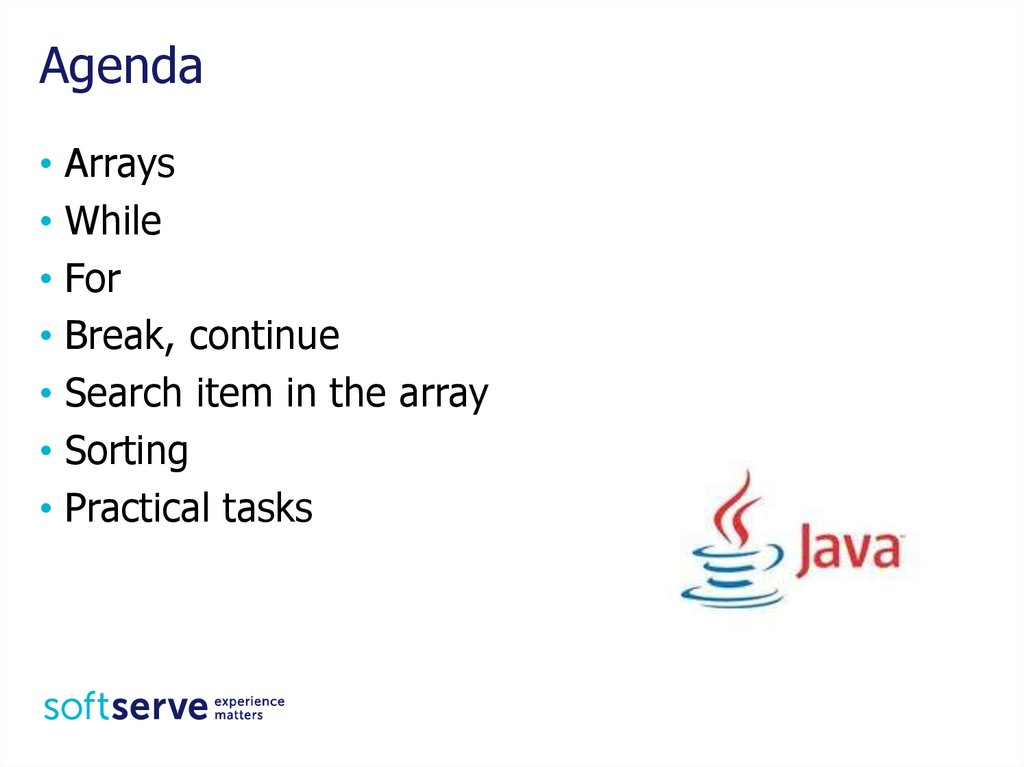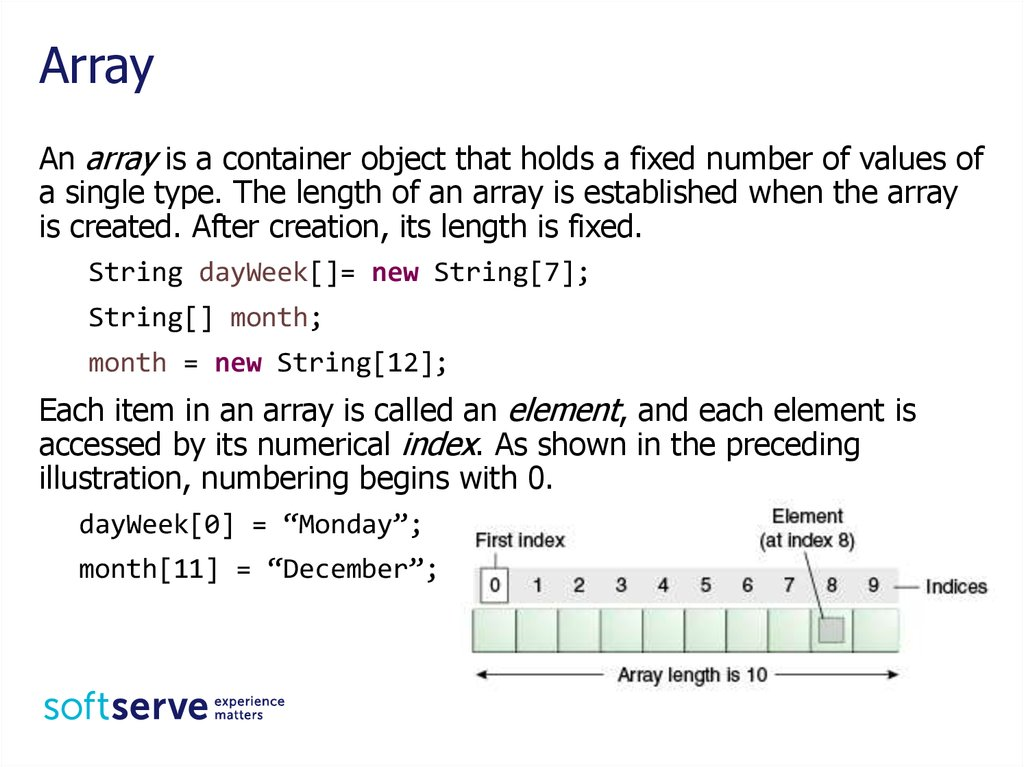# Arrays Loops. Java Core

Java Core
Arrays
Loops
IT Academy
04/2016

## 2. Agenda

• Arrays
• While
• For
• Break, continue
• Search item in the array
• Sorting
• Practical tasks

## 3. Array

An array is a container object that holds a fixed number of values of
a single type. The length of an array is established when the array
is created. After creation, its length is fixed.
String dayWeek[]= new String;
String[] month;
month = new String;
Each item in an array is called an element, and each element is
accessed by its numerical index. As shown in the preceding
illustration, numbering begins with 0.
dayWeek = “Monday”;
month = “December”;

## 4. Array

int month_days[ ]
= {31, 28, 31, 30, 31, 30,
31, 31, 30, 31, 30, 31} ;
int month_days[ ] = new int;
month_days = 31;
month_days = 28;
// . . .
month_days = 31;
int n = month_days.length;
System.out.println(month);
// n = 12
//[[email protected]
Need override toString();
or use Arrays.toString(month);

## 5. Array

char twod1[ ][ ]= new char;
char[ ][ ] twod2= new char;
double m[ ][ ]=
{{0, 1, 2, 3},
{4, 5, 6, 7},
{8, 9, 10, 11},
{12, 13, 14, 15}};
int twoD[ ][ ]= new int [ ];
twoD= new int ;
double m;
// Error;
twoD= new int ;
twoD= new int ;
twoD= new int ;
int[ ][ ] irregular={{1},{2,3,4},{5},{6,7}}; // Rows not equal

## 6. while

A while loop statement repeatedly executes a target statement as long
as a given condition is true.
while (condition){
statements;
}
What will be displayed?
int number = 0;
while (number <= 5) {
System.out.println(number);
number++;
}

## 7. do while

The difference between do-while and while is that do-while evaluates
its expression at the bottom of the loop instead of the top. Therefore,
the statements within the do block are always executed at least once
do {
statements;
} while (condition);
What will be displayed?
int i = 0;
do {
System.out.print(++i);
} while (i < 5);

## 8. for

The for statement provides a compact way to iterate over a range of
values.
for (init; condition ; increment) {
statement(s);
}
What will be displayed?
int[] month = {5, 6, 8, 3, 5, 7, 9};
for(int i = 0; i < month.length; i++){
System.out.println(month[i]);
}

## 9. for

Another representation of statement for
for (type variable : collection) {
statement(s);
}
int[] workHours = { 8, 6, 8, 7, 7 };
for (int h = 0; h < workHours.length; h++) {
System.out.println(workHours[h]);
}
or
for (int h : workHours) {
System.out.println(h);
}

## 10. break

The break statement terminates the for, while and do-while loop.
What will be displayed?
Scanner sc = new Scanner(System.in);
int n = 0;
for (int i = 0; i < 5; i++) {
System.out.println("Input number");
n = Integer.parseInt(sc.nextLine());
if (n < 0){
n = i;
break;
}
}
System.out.println(n);
sc.close();

## 11. continue

The continue statement skips the current iteration
the for, while and do-while loop.
What will be displayed?
Scanner sc = new Scanner(System.in);
int sum = 0;
int n;
for (int i = 0; i < 5; i++) {
System.out.println("Input number");
n = Integer.parseInt(sc.nextLine());
if (n < 0){
n = i;
continue;
}
sum += n;
}
System.out.println(sum);
sc.close();

## 12. Sum, product, amount

There’s an array int[] arr = {2, -5, 7, -4, 8};
What will results after running next code?
int sum = 0;
for (int i = 0; i < arr.length; i++) { sum += arr[i];}
System.out.println("Sum = " + sum);
int product = 1;
for (int i = 0; i < 5; i++) {
if (arr[i] > 0) {product = product * arr[i];}}
System.out.println("Product = " + product);
int amount = 0;
for (int a : arr) {
if (a > 0 && a <= 7) { amount++; }
}
System.out.println("Amount = " + amount);

## 13. Minimum, maximum ...

There’s an array int[] arr = {2, -5, 7, -4, 8};
What will results after running next code?
int max = arr;
int imax = 0;
int i = 0;
while (i < arr.length) {
if (arr[i] > max) {
max = arr[i];
imax = i;
}
i++;
}
System.out.print("Maximum = " + max);
System.out.println(" is in " + (imax + 1) + " place");

## 14. Sorting

There’s an array int[] arr = {2, -5, 7, -4, 8};
What will results after running next code?
int tmp;
for (int i = 0; i < arr.length - 1; i++) {
for (int j = i + 1; j < arr.length; j++) {
if (arr[i] < arr[j]) {
tmp = arr[i];
arr[i] = arr[j];
arr[j] = tmp;
}
}
}
for (int i = 0; i < arr.length; i++) {
System.out.println(arr[i]);
}

## 15. Practical tasks

1. Create an array of ten integers. Display
• the biggest of these numbers;
• the sum of positive numbers in the array;
• the amount of negative numbers in the array.
What values there are more: negative or positive?
2. Create a class Employee with fields name, department number,
salary. Create five objects of class Employee. Display
• all employees of a certain department (enter department
number in the console);
• arrange workers by the field salary in descending order.

## 16. HomeWork (online course)

• UDEMY course "Java Tutorial
for Complete Beginners":
https://www.udemy.com/java
-tutorial/
• Complete lessons 8, 9, 12, 14
- 16:

## 17. Homework

1. Ask user to enter the number of month. Read the value and write the
amount of days in this month (create array with amount days of each
month).
2. Enter 10 integer numbers. Calculate the sum of first 5 elements if they
are positive or product of last 5 element in the other case.
3. Enter 5 integer numbers. Find
• position of second positive number;
• minimum and its position in the array.
4. Organize entering integers until the first negative number. Count the
product of all entered even numbers.
5. Create class Car with fields type, year of production and engine
capacity. Create and initialize four instances of class Car. Display cars
• certain model year (enter year in the console);
• ordered by the field year.

## 18. THE END

USA HQ
Toll Free: 866-687-3588
Tel: +1-512-516-8880
Ukraine HQ
Tel: +380-32-240-9090
Bulgaria
Tel: +359-2-902-3760
Poland
Tel: +48-71-382-2800
EMAIL
[email protected]
Germany
Tel: +49-69-2602-5857
UK
Tel: +44-207-544-8414
WEBSITE:
www.softserveinc.com
Netherlands
Tel: +31-20-262-33-23